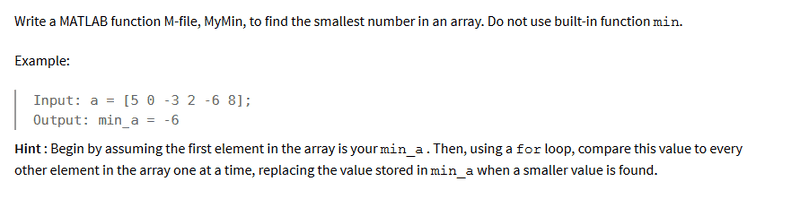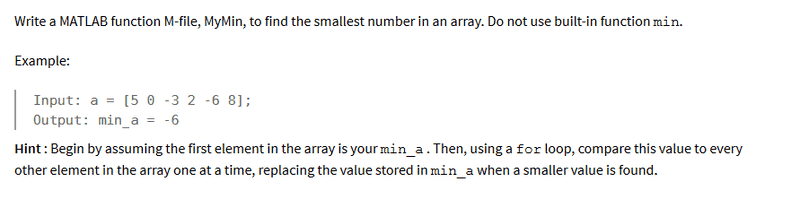# MATLAB "for loops"

## Homework Statement-

## The Attempt at a Solution

I've been trying several different ways but I just can't seem to figure this one out. It seems to me like I will need nested for loops, which we haven't learned yet. The way I read the question is that this program needs to be able to solve any arbitrary matrix, which means that it can have x rows and y columns. So I am completely perplexed.

I tried writing a for loop that extracts all the rows into one row:
-----------
clc;clear

a=input('enter a matrix: ')
HowBig=size(a);
rows=HowBig(1,1);
columns=HowBig(1,2);

for j=1:rows
a(j)=[a(j,:)]
end
----------------

But matlab refuses to run this code because

In an assignment A(I) = B, the number of elements in B and I must be the same.

after running this program I wanted to do another loop like this:

-----------------------------------------
for i=1:length(a)
if i==length(a)
break
end

if a(
1,i)<=a(1,i+1)
min_a=a(1,i);
else
min_a = a(1,i+1);
end %if

end %for

disp(num2str(min_a))
------------------------------------------

Obviously something is really wrong here but I am completely stuck. The second line of code seems to run perfectly well, I just cannot figure out what to do with the first part. Is there any way to pull out the whole Matrix into a single row vector?

## Answers and Replies

Simon Bridge
Science Advisor
Homework Helper
I don't think you need to nest for loops for this.
What you do need to do is work out the logic in advance.

Testing:
Code:
> a=magic(3)
a =

8   1   6
3   5   7
4   9   2
... the magic squares are useful test matrixes.

Lets try out that first instruction, pretend that j=1 for starters.
Code:
[/SIZE]
> a(1)=[a(1,:)]
error: A(I) = X: X must have the same size as I
... well yes, naturally, because
Code:
> size(1)
ans =

1   1

> size(a(1,:))
ans =

1   3
... what were you trying to do?
Bearing in mind that you had already used "a" as the input matrix ... assigning a(something)=something-else will change the matrix.
Probably best not to do that.

I don't follow your reasoning for the second set of code.
Sart by working out the logic of the program - go through what the program has to do one step at a time.

•Feodalherren
Mark44
Mentor

## Homework Statement-

## The Attempt at a Solution

I've been trying several different ways but I just can't seem to figure this one out. It seems to me like I will need nested for loops, which we haven't learned yet. The way I read the question is that this program needs to be able to solve any arbitrary matrix, which means that it can have x rows and y columns. So I am completely perplexed.

I tried writing a for loop that extracts all the rows into one row:
-----------
clc;clear

a=input('enter a matrix: ')
HowBig=size(a);
rows=HowBig(1,1);
columns=HowBig(1,2);

for j=1:rows
a(j)=[a(j,:)]
end
----------------

But matlab refuses to run this code because

In an assignment A(I) = B, the number of elements in B and I must be the same.
You're really off track here. You are supposed to be working with an array, which is a matrix with one row. In your 3rd line of code you are setting HowBig to the singel value returned by size, but in your 4th and 5th line, you're assuming that HowBig is a two-dimensional array.

There is no need for doing anything with rows and columns as you're doing in the 4th and 5th lines.

The basic algorithm should be something like this:
Code:
Initialize an array with some numbers.
Set min_a to arr(1)
For each element in the array
if arr(i) < min_a
min_a = arr(i)
end if
end for
Feodalherren said:
after running this program I wanted to do another loop like this:

-----------------------------------------
for i=1:length(a)
if i==length(a)
break
end

if a(
1,i)<=a(1,i+1)
min_a=a(1,i);
else
min_a = a(1,i+1);
end %if

end %for

disp(num2str(min_a))
------------------------------------------

Obviously something is really wrong here but I am completely stuck. The second line of code seems to run perfectly well, I just cannot figure out what to do with the first part. Is there any way to pull out the whole Matrix into a single row vector?

•Feodalherren
------------------
L=length(a);

min_a=a(1,1);

for i=1:L
if a(1,i) <= min_a
min_a = a(1,i);
end
end

min_a

------------------

This worked for the most part. But even though the problem says array, my code failed one test with this message:

----------------
Fail
%%
A = [5 2 4;-6 7 -10;4 -4 8];
assert(MyMin(A)==-10,'Failed matrix test')

------------------

So it looks like I do indeed have to adjust for matrices which brings me back to my initial problem.
As for what I was trying to do: I was trying to figure out a way of testing ALL values in a matrix against each other.

Mark44
Mentor
------------------
L=length(a);

min_a=a(1,1);

for i=1:L
if a(1,i) <= min_a
min_a = a(1,i);
end
end

min_a

------------------

This worked for the most part. But even though the problem says array, my code failed one test with this message:

----------------
Fail
%%
A = [5 2 4;-6 7 -10;4 -4 8];
assert(MyMin(A)==-10,'Failed matrix test')

------------------

So it looks like I do indeed have to adjust for matrices which brings me back to my initial problem.
As for what I was trying to do: I was trying to figure out a way of testing ALL values in a matrix against each other.
Why are you using a 2-D array? You are making it more complicated than it needs to be. The problem statement uses a 1-D array.

I'm not using it. I plug my code into a thing called Cody and it checks for me. I'm guessing my professor made a mistake in the problem statement as Cody does check for matrices. So Cody plugged in the 2D array.

Mark44
Mentor
I don't know anything about Cody - what it is or what it does. Can't you write your matlab code without Cody? How can the problem statement be wrong? It is what it is, and the example input is a one-D array. You can use any text editor, like NotePad or NotePad++ to write an M-file.

Mark44
Mentor
This should work.
Code:
a = [5 0 -3 2 -6 8]
L=length(a);
min_a=a(1);

for i=2:L
if a(i) <= min_a
min_a = a(i);
end
end

min_a;

After this code runs, it should display -6.

Since your task is to write an M-file, you need to write a function that returns the smallest value in the array.

Cody is a program that tests MATLAB codes. It's automatic. You submit a code online and Cody tests it and lets you know which tests you passed and didn't pass. The problems for Cody are written by the professor who also does the tests. In this case there was a mistake in the problem statement.